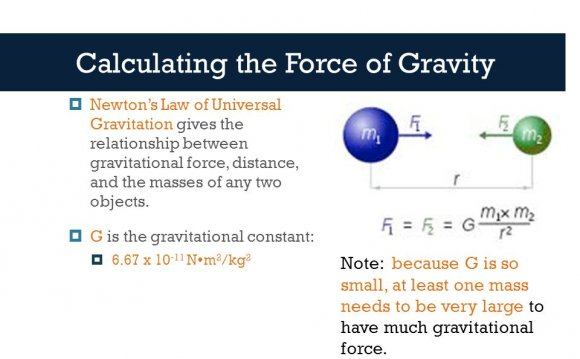# Calculating the force of gravity

August 29, 2016The weight of an object is given by W=mg, the force of gravity, which comes from the law of gravity at the surface of the Earth in the inverse square law form:

At standard sea level, the acceleration of gravity has the value g = 9.8 m/s2, but that value diminishes according to the inverse square law at greater distances from the earth. The value of g at any given height, say the height of an, can be calculated from the above expression.

Please note that the above calculation gives the correct value for the acceleration of gravity only for positive values of h, i.e., for points outside the Earth. If you drilled a hole through the center of the Earth, the acceleration of gravity would decrease with the radius on the way to the center of the Earth. If the Earth were of uniform density (which it is not!), the acceleration of gravity would decrease linearly to half the surface value of g at half the radius of the Earth and approach zero as you approached the center of the Earth.

Source: hyperphysics.phy-astr.gsu.edu